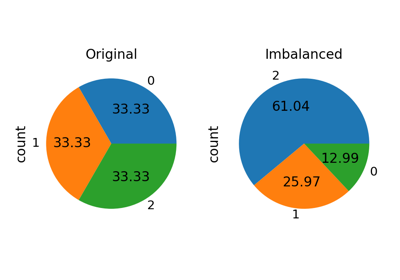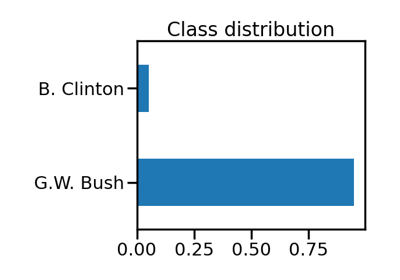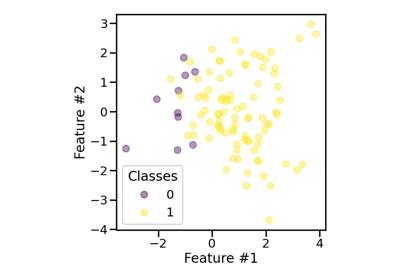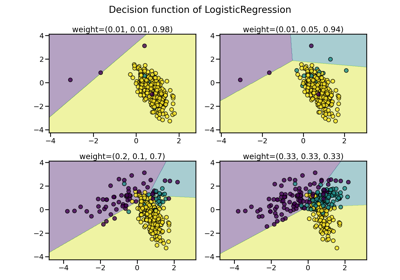# RandomOverSampler#

class imblearn.over_sampling.RandomOverSampler(*, sampling_strategy='auto', random_state=None, shrinkage=None)[source]#

Class to perform random over-sampling.

Object to over-sample the minority class(es) by picking samples at random with replacement. The bootstrap can be generated in a smoothed manner.

Read more in the User Guide.

Parameters:
sampling_strategyfloat, str, dict or callable, default=’auto’

Sampling information to resample the data set.

• When float, it corresponds to the desired ratio of the number of samples in the minority class over the number of samples in the majority class after resampling. Therefore, the ratio is expressed as $$\alpha_{os} = N_{rm} / N_{M}$$ where $$N_{rm}$$ is the number of samples in the minority class after resampling and $$N_{M}$$ is the number of samples in the majority class.

Warning

float is only available for binary classification. An error is raised for multi-class classification.

• When str, specify the class targeted by the resampling. The number of samples in the different classes will be equalized. Possible choices are:

'minority': resample only the minority class;

'not minority': resample all classes but the minority class;

'not majority': resample all classes but the majority class;

'all': resample all classes;

'auto': equivalent to 'not majority'.

• When dict, the keys correspond to the targeted classes. The values correspond to the desired number of samples for each targeted class.

• When callable, function taking y and returns a dict. The keys correspond to the targeted classes. The values correspond to the desired number of samples for each class.

random_stateint, RandomState instance, default=None

Control the randomization of the algorithm.

• If int, random_state is the seed used by the random number generator;

• If RandomState instance, random_state is the random number generator;

• If None, the random number generator is the RandomState instance used by np.random.

shrinkagefloat or dict, default=None

Parameter controlling the shrinkage applied to the covariance matrix. when a smoothed bootstrap is generated. The options are:

• if None, a normal bootstrap will be generated without perturbation. It is equivalent to shrinkage=0 as well;

• if a float is given, the shrinkage factor will be used for all classes to generate the smoothed bootstrap;

• if a dict is given, the shrinkage factor will specific for each class. The key correspond to the targeted class and the value is the shrinkage factor.

The value needs of the shrinkage parameter needs to be higher or equal to 0.

New in version 0.8.

Attributes:
sampling_strategy_dict

Dictionary containing the information to sample the dataset. The keys corresponds to the class labels from which to sample and the values are the number of samples to sample.

sample_indices_ndarray of shape (n_new_samples,)

Indices of the samples selected.

New in version 0.4.

shrinkage_dict or None

The per-class shrinkage factor used to generate the smoothed bootstrap sample. When shrinkage=None a normal bootstrap will be generated.

New in version 0.8.

n_features_in_int

Number of features in the input dataset.

New in version 0.9.

feature_names_in_ndarray of shape (n_features_in_,)

Names of features seen during fit. Defined only when X has feature names that are all strings.

New in version 0.10.

BorderlineSMOTE

Over-sample using the borderline-SMOTE variant.

SMOTE

Over-sample using SMOTE.

SMOTENC

Over-sample using SMOTE for continuous and categorical features.

SMOTEN

Over-sample using the SMOTE variant specifically for categorical features only.

SVMSMOTE

Over-sample using SVM-SMOTE variant.

ADASYN

KMeansSMOTE

Over-sample applying a clustering before to oversample using SMOTE.

Notes

Supports multi-class resampling by sampling each class independently. Supports heterogeneous data as object array containing string and numeric data.

When generating a smoothed bootstrap, this method is also known as Random Over-Sampling Examples (ROSE) .

Warning

Since smoothed bootstrap are generated by adding a small perturbation to the drawn samples, this method is not adequate when working with sparse matrices.

References



G Menardi, N. Torelli, “Training and assessing classification rules with imbalanced data,” Data Mining and Knowledge Discovery, 28(1), pp.92-122, 2014.

Examples

>>> from collections import Counter
>>> from sklearn.datasets import make_classification
>>> from imblearn.over_sampling import RandomOverSampler
>>> X, y = make_classification(n_classes=2, class_sep=2,
... weights=[0.1, 0.9], n_informative=3, n_redundant=1, flip_y=0,
... n_features=20, n_clusters_per_class=1, n_samples=1000, random_state=10)
>>> print('Original dataset shape %s' % Counter(y))
Original dataset shape Counter({1: 900, 0: 100})
>>> ros = RandomOverSampler(random_state=42)
>>> X_res, y_res = ros.fit_resample(X, y)
>>> print('Resampled dataset shape %s' % Counter(y_res))
Resampled dataset shape Counter({0: 900, 1: 900})


Methods

 fit(X, y) Check inputs and statistics of the sampler. fit_resample(X, y) Resample the dataset. get_feature_names_out([input_features]) Get output feature names for transformation. Get metadata routing of this object. get_params([deep]) Get parameters for this estimator. set_params(**params) Set the parameters of this estimator.
fit(X, y)[source]#

Check inputs and statistics of the sampler.

You should use fit_resample in all cases.

Parameters:
X{array-like, dataframe, sparse matrix} of shape (n_samples, n_features)

Data array.

yarray-like of shape (n_samples,)

Target array.

Returns:
selfobject

Return the instance itself.

fit_resample(X, y)[source]#

Resample the dataset.

Parameters:
X{array-like, dataframe, sparse matrix} of shape (n_samples, n_features)

Matrix containing the data which have to be sampled.

yarray-like of shape (n_samples,)

Corresponding label for each sample in X.

Returns:
X_resampled{array-like, dataframe, sparse matrix} of shape (n_samples_new, n_features)

The array containing the resampled data.

y_resampledarray-like of shape (n_samples_new,)

The corresponding label of X_resampled.

get_feature_names_out(input_features=None)[source]#

Get output feature names for transformation.

Parameters:
input_featuresarray-like of str or None, default=None

Input features.

• If input_features is None, then feature_names_in_ is used as feature names in. If feature_names_in_ is not defined, then the following input feature names are generated: ["x0", "x1", ..., "x(n_features_in_ - 1)"].

• If input_features is an array-like, then input_features must match feature_names_in_ if feature_names_in_ is defined.

Returns:
feature_names_outndarray of str objects

Same as input features.

Get metadata routing of this object.

Please check User Guide on how the routing mechanism works.

Returns:

A MetadataRequest encapsulating routing information.

get_params(deep=True)[source]#

Get parameters for this estimator.

Parameters:
deepbool, default=True

If True, will return the parameters for this estimator and contained subobjects that are estimators.

Returns:
paramsdict

Parameter names mapped to their values.

set_params(**params)[source]#

Set the parameters of this estimator.

The method works on simple estimators as well as on nested objects (such as Pipeline). The latter have parameters of the form <component>__<parameter> so that it’s possible to update each component of a nested object.

Parameters:
**paramsdict

Estimator parameters.

Returns:
selfestimator instance

Estimator instance.

## Examples using imblearn.over_sampling.RandomOverSampler#How to use sampling_strategy in imbalanced-learn

How to use sampling_strategy in imbalanced-learnBenchmark over-sampling methods in a face recognition task

Benchmark over-sampling methods in a face recognition taskBagging classifiers using sampler

Bagging classifiers using samplerEffect of the shrinkage factor in random over-sampling

Effect of the shrinkage factor in random over-samplingCompare over-sampling samplers

Compare over-sampling samplers